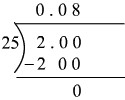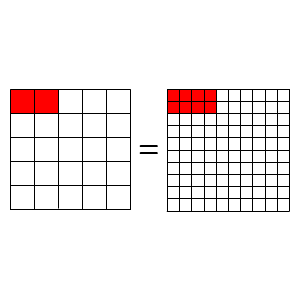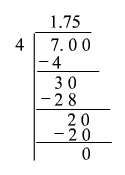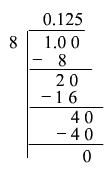# Converting Fractions to Percent

Remember that a percent is really just a special way of expressing a fraction as a number out of $100$ .

To convert a fraction to a percent, first divide the numerator by the denominator. Then multiply the decimal by $100$ .

That is, the fraction $\frac{4}{8}$ can be converted to decimal by dividing $4$ by $8$ . It can be converted to percent by multiplying the decimal by $100$ .

$\begin{array}{l}4÷8=0.5\\ 0.5×100=50\end{array}$

So, the fraction $\frac{4}{8}$ is equivalent to $50%$ .

Example 1:

Write $\frac{2}{25}$ as a percent.

Since $25$ is larger than $2$ , in order to divide, we must add a decimal point and some zeroes after the $2$ .  We may not know how many zeroes to add but it doesn't matter.  If we add too many we can erase the extras; if we don't add enough, we can add more.So,

$\begin{array}{l}\frac{2}{25}=0.08\\ 0.08×100=8\end{array}$

Therefore, the fraction $\frac{2}{25}$ is equivalent to $8%$ .

Look at the image below, it shows that the fraction $\frac{2}{25}$ is same as $8$ out of $100$ , that is, $8%$ .Example 2:

Write $\frac{7}{4}$ as a percent.

Divide $7$ by $4$ .So,

$\begin{array}{l}\frac{7}{4}=1.75\\ 1.75×100=175\end{array}$

Therefore, the fraction $\frac{7}{4}$ is equivalent to $175%$ .

Example 3:

Write $\frac{1}{8}$ as a percent.

Divide $1$ by  .So,

$\begin{array}{l}\frac{1}{8}=0.125\\ 0.125×100=12.5\end{array}$

Therefore, the fraction $\frac{1}{8}$ is equivalent to $12.5%$ .